Home > ACC7 > Chapter cc39 > Lesson cc39.2.1 > Problem9-64

9-64.
1.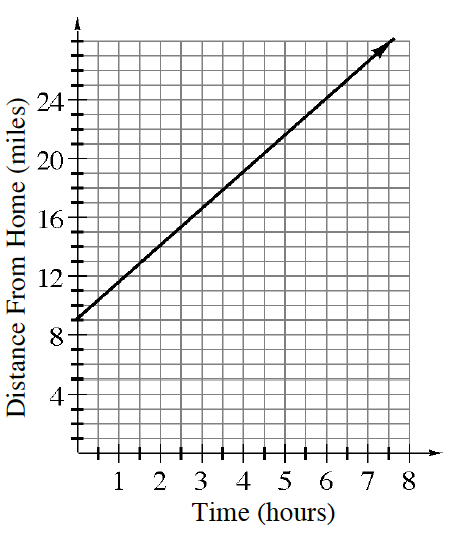Use the graph at right to answer the following questions. Homework Help ✎

1. What kind of growth does this graph show? How do you know?

2. What is this graph describing? Write an appropriate title for the graph.

3. How far from home is the person when the graph starts?

4. How fast is the person traveling? Explain how you can use the graph to determine the rate of travel.

5. Write an equation to represent the line on the graph.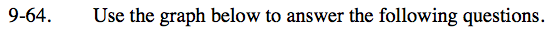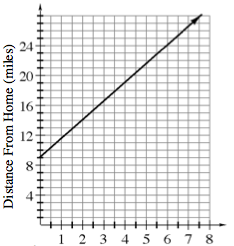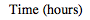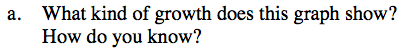Does the graph show linear growth or non-linear growth? You can tell because linear growth creates a line.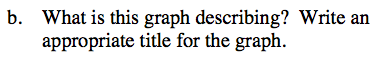Look at the x-axis and y-axis labels. The graph shows the distance from home at several different time periods.

Possible title: Distance and Time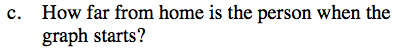The graph starts at 0 hours. Which means this person just started to travel. What is the distance from home at 0 hours?

He starts at 9 miles from home.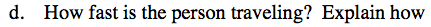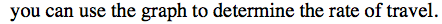Find the slope of the line.

$\frac{10}{4} = 2.5\text{ miles per\;hour}$

$\text{Find the \frac{rise}{run}.}$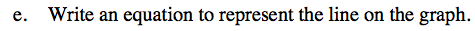A slope of 2.5 means that the distance from home increases 2.5 miles per hour. That means we multiply 2.5 and any hour which we will call x.

y = 2.5x + 9
2.5 relates to amount traveled every hour and 9 relates to the starting point.

Since we know how fast this person is traveling, we also need to know the starting distance. From part (b) we found that this person started 9 miles away from home.